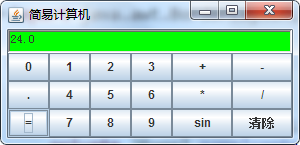1.简单的运算：（以加法为例：123+456）

2.倒数的运算：（以123为例）

3.退格的运算：（以123为例）

4.正负号的运算：（以123为例）

4个回答

1.架想，是Java弹窗版本还是网页应用版本
2.设计，设计计算机的样式
3.算法和传值
4.挺容易的，加油Java计算器的完整代码

Java语言如何模拟一个简单的计算器程序，用eclpse工具的步骤？

Java语言如何模拟一个简单的计算器程序，用eclpse工具的步骤？Java语言如何模拟一个简单的计算器程序，用eclpse工具的步骤？

JAVA课程设计实数计算器求指导思路

【问题描述】运用面向对象程序设计知识，利用Java语言设计和实现一个复数计算器。要求具备如下主要功能： （1）建立实数类、复数类 （2）实现实数、复数信息的初始化 （3）实现实数的加、减、乘、除、自增、自减、求平方、二次方根等操作 （4）实现复数的加、减、乘、除、取模、求平方、求共轭复数、求单个复数的向量角、求两个复数的夹角等运算 （5）实现实数、复数信息的输出 在实现过程中，需利用面向对象程序设计理论的基础知识，充分体现出Java语言关于类、继承、封装与多态等核心概念，每一个类应包含数据成员和成员函数。 我的问题是：为什么要建2个类，这两个类改设置些什么属性？怎么在主函数里面用？以及如何求单个复数向量角

java大作业:多功能计算器，请大神们指教！

java制作计算器功能遇到的问题

import java.util.Scanner; class demo2 { public static void main(String[] args) { Scanner scanner = new Scanner(System.in); System.out.println("请选择你要使用的计算器功能："); System.out.println("1：加 2：减 3：乘 4：除 5：平方"); int n = scanner.nextInt(); /*1*/ if (n =1) { System.out.println("请输入第一个数据(必须遵循算数规则）："); long num1 = scanner.nextInt(); System.out.println("请输入第二个数据："); long num2 = scanner.nextInt(); System.out.println("所得的结果为:"+(num1+num2)); return; } /*2*/ if (n>1 || n<3) { System.out.println("请输入第一个数据(必须遵循算数规则）："); long num1 = scanner.nextInt(); System.out.println("请输入第二个数据："); long num2 = scanner.nextInt(); System.out.println("所得的结果为:"+(num1-num2)); return; } /*3*/ if (n>2 || n<4) { System.out.println("请输入第一个数据(必须遵循算数规则）："); long num1 = scanner.nextInt(); System.out.println("请输入第二个数据："); long num2 = scanner.nextInt(); System.out.println("所得的结果为:"+(num1*num2)); return; } /*4*/ if (n>3 || n<5) { System.out.println("请输入第一个数据(必须遵循算数规则）："); long num1 = scanner.nextInt(); System.out.println("请输入第二个数据："); long num2 = scanner.nextInt(); System.out.println("所得的结果为:"+(num1/num2)); return; } /*5*/ if (n>4) { System.out.println("请输入平方数据(必须遵循算数规则）："); long num1 = scanner.nextInt(); System.out.println("所得的结果为:"+(num1*num1)); return; } } } 这是打算做一个计算器的功能，遇到了3个问题 1：通过输入数字选择计算器功能（1、加法 2、减法 。。。），我想用if（n=1）,if(n=2) 这种的，但是报错：错误: 不兼容的类型: int无法转换为boolean /*1*/ if (n =1) ^ 所以只能用了n<2表示n=1... > 2:从选择乘法开始，也就是n=3(n>2 || n<4)时开始，计算的结果是减法，选择除法也是 > 3: 我想做到输入数据算出结果后，不是直接结束，而是跳转到最前面，继续重新选择计算器功能 求大神解惑，新学java，懂得很少，问题也比较多

java 实现计算器功能

java简易计算器实现，添加能够切换简易计算器和科学计算器的按键。

Python 中的GUI写一个复杂的计算器程序

java简单计算器，清除，回退和等号功能

JAVA 计算器，求大神，急急急，帮帮忙

import java.awt.Frame; import java.awt.Panel; import java.awt.GridLayout; import java.awt.FlowLayout; import java.awt.*; import java.awt.event.*; import java.util.Enumeration; import java.util.Stack; import java.lang.Integer; public class ComputerFrame extends Frame implements ActionListener { Panel numberPanel; TextField text; Panel operatorPanel; Button[] num; Button[] oper; public ComputerFrame() { super("这是一个计算机"); init(); } public void init() { operatorPanel=new Panel(); numberPanel=new Panel(); text=new TextField(15); num=new Button; oper=new Button; oper=new Button("C"); oper=new Button("←"); oper=new Button("+"); oper=new Button("-"); oper=new Button("*"); oper=new Button("/"); oper=new Button("="); setSize(200,500); setFont(new Font("Dialog",Font.PLAIN,30)); setLayout(new FlowLayout()); numberPanel.setLayout(new GridLayout(4,3,5,5)); operatorPanel.setLayout(new GridLayout(4,2,5,5)); add(text); add(numberPanel); add(operatorPanel); for(int i=1;i<10;i++) { num[i]=new Button(String.valueOf(i)); numberPanel.add(num[i]); num[i].addActionListener(this); } num=new Button(String.valueOf(0)); numberPanel.add(num); num.addActionListener(this); for(int i=0;i<=6;i++) { operatorPanel.add(oper[i]); oper[i].addActionListener(this); } } public void actionPerformed(ActionEvent e) { String t=text.getText(); int len=t.length(); int[] nums=new int; String oparator=""; for(int i=0;i<=9;i++) { if(e.getSource()==num[i]) text.setText(t+num[i].getLabel()); } for(int i=2;i<6;i++) { if(e.getSource()==oper[i]) {text.setText(t+oper[i].getLabel()); oparator=oper[i].getLabel(); } } //清空 if(e.getSource()==oper) { text.setText(null); } //删去 if(e.getSource()==oper) { text.setText(t.substring(0,len-1)); } //等于 if(e.getSource()==oper) { text.setText(this.calculator(t)); } } public String calculator(String t){ char lastoper='='; char c; char operator; int num1; int num2; String num=" "; String result; Stack operstack=new Stack(); Stack numstack=new Stack(); operstack.push(new Character('=')); numstack.push(new Character('0')); for(int i=0;i <t.length();i++) { c=t.charAt(i); //情况一 数字 if(Character.isDigit(c)) { num=num+String.valueOf(c); } //情况二 运算符 else if((c=='+')||(c=='-')||(c=='*')||(c=='/')||(c=='=')) { if(!num.equals(" ")) { numstack.push(new Integer(num)); num=""; char s=((Character)operstack.peek()).charValue(); while(getRual(s)>getRual(c))//比较优先权 { operator=((Character)operstack.pop()).charValue(); num1=((Integer)numstack.pop()).intValue(); num2=((Integer)numstack.pop()).intValue(); numstack.push(cal(num1,num2,operator)); } if(getRual(s)<=getRual(c)) { operstack.push(new Character(c)); lastoper=c; continue; } } } } Enumeration items=operstack.elements(); while(items.hasMoreElements()) { operator=((Character)operstack.pop()).charValue(); num1=((Integer)numstack.pop()).intValue(); num2=((Integer)numstack.pop()).intValue(); numstack.push(cal(num1,num2,operator)); } result=((Integer)operstack.pop()).toString(); return result; } public static int getRual(char c) { switch(c) { case '+':return 1; case '-':return 1; case '*':return 2; case '/':return 2; case '=':return 0; default: return -1; } } public static int cal(int num1,int num2,char c) { switch(c) { case '+':return num1+num2; case '-':return num1-num2; case '*':return num1*num2; case '/':return num1/num2; default: return -1; } } public static void main(String args[]) { ComputerFrame demo=new ComputerFrame(); demo.setSize(300,400); demo.show(); }

MySQL数据库面试题（2020最新版）

HashMap底层实现原理，红黑树，B+树，B树的结构原理 Spring的AOP和IOC是什么？它们常见的使用场景有哪些？Spring事务，事务的属性，传播行为，数据库隔离级别 Spring和SpringMVC，MyBatis以及SpringBoot的注解分别有哪些？SpringMVC的工作原理，SpringBoot框架的优点，MyBatis框架的优点 SpringCould组件有哪些，他们...

Python爬虫，高清美图我全都要（彼岸桌面壁纸）

6年开发经验女程序员，面试京东Java岗要求薪资28K

Java岗开发3年，公司临时抽查算法，离职后这几题我记一辈子